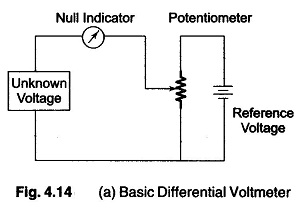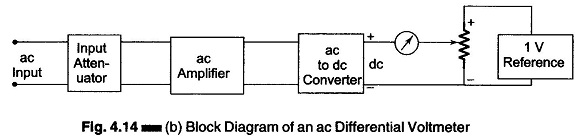## Differential Voltmeter:

The Differential Voltmeter technique, is one of the most common and accurate methods of measuring unknown voltages. In this technique, the voltmeter is used to indicate the difference between known and unknown voltages, i.e., an unknown voltage is compared to a known voltage.

Figure 4.14 (a) shows a basic circuit of a differential voltmeter based on the potentiometric method; hence it is sometimes also called a potentiometric volt­meter.In this method, the potentiometer is varied until the voltage across it equals the unknown voltage, which is indicated by the null indicator reading zero. Under null conditions, Voltage the meter draws current from neither the reference source nor the un-known voltage source, and hence the differential voltmeter presents an infinite impedance to the unknown source. (The null meter serves as an indicator only.)

To detect small differences the meter movement must be sensitive, but it need not be calibrated, since only zero has to be indicated.

The reference source used is usually a 1 V dc standard source or a zener controlled precision supply. A high voltage reference supply is used for measuring high voltages.

The usual practice, however, is to employ voltage dividers or attenuators across an unknown source to reduce the voltage. The input voltage divider has a relatively low input impedance, especially for unknown voltages much higher than the reference standard. The attenuation will have a loading effect and the input resistance of voltmeter is not infinity when an attenuator is used.In order to measure ac voltages, the ac voltage must be converted into dc by incorporating a precision rectifier circuit. A block diagram of an ac differential voltmeter is shown in Fig. 4.14 (b).

Scroll to Top